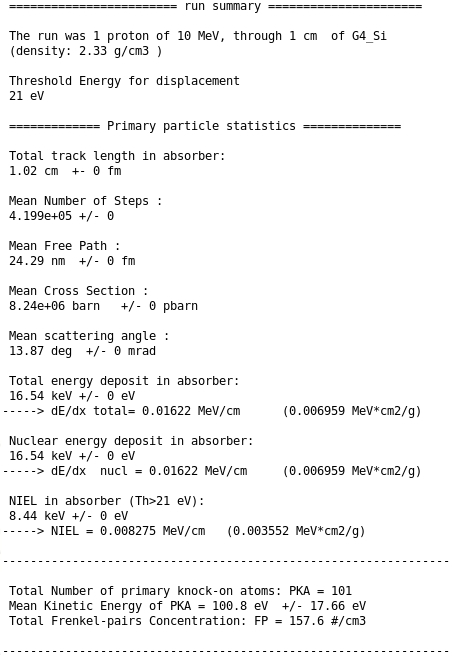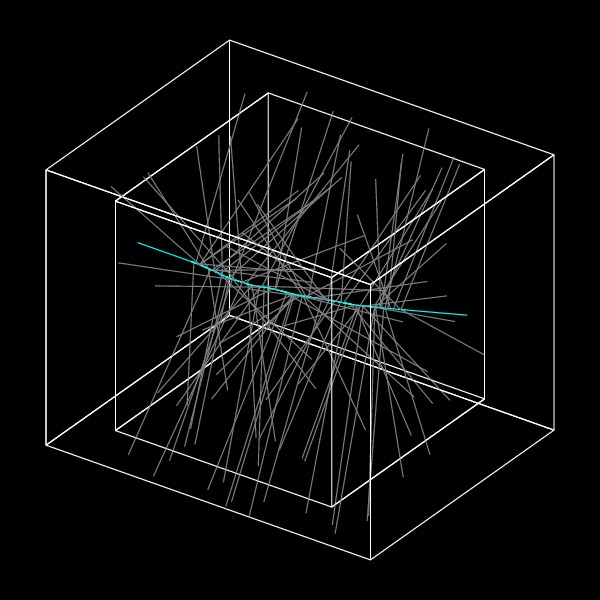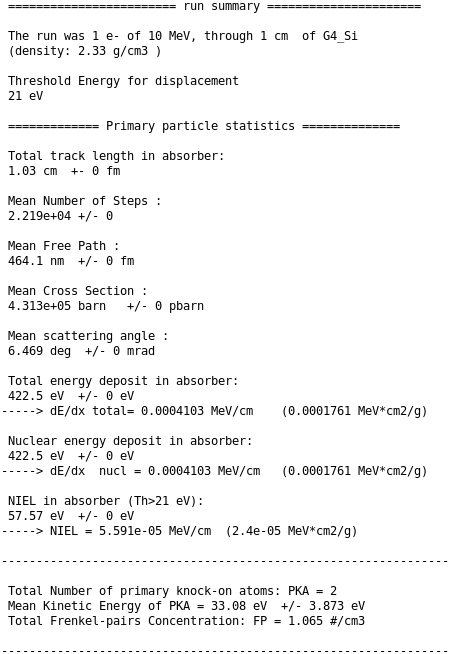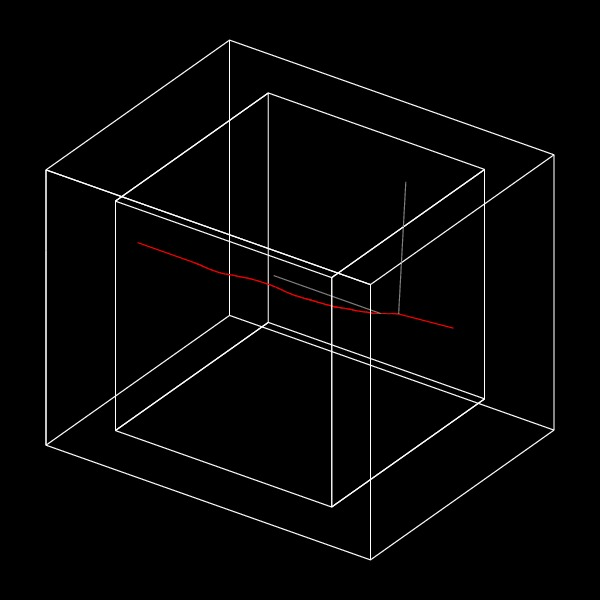### test58

test58 is the reference example for NIEL and nuclear stopping power calculation in Geant4.

The physical values returned, computed as an average on the number of impinging particles, are:

• Total track lenght:
X0
is the effective lenght of the path traversed by the incoming particle inside the absorber.

• Mean Number of Step:
NS is the number of interactions that were produced along the path.

• Mean free path:
λ=X0/NS is mean path traversed in between two consecutive interactions.

• Interaction Cross Section:
σ=(λ*n)-1 where n is the number density of the target material.

• Mean scattering angle:
is the total deflection angle out of the detector.

• Total Energy deposited:
is the energy deposited by means of the physics process that are defined in the physic list (ionization, displacement...).

• Nuclear Recoil Energy deposited:
is the energy deposited by nuclear recoil per unit lenght (MeV cm-1) - or (MeV cm2 g-1) - .

• NIEL:
is the Non Ionizing Energy Loss computed with the selected threshold energy.

• Total Number of primary knock-on atoms (PKA):
is the number of displaced atoms.

• Mean Kinetic Energy of PKA

• Total Frenkel-pairs concentration:
FP=Edis/(2.5*Th) is the concentration of stable defects, where Edis is the energy that goes into displacement and Th is the selected threshold energy for displacement.

Two examples, with protons and electrons as incident particles are provided.

### Protons

One single proton of 10 MeV energy is impinging on a Silicon cube (1x1x1 cm3). The threshold energy for displacement is set to 21 eV. The only physical process selected in the physic list is the single coulomb scattering. The output result is given:

####The visualization of the event is also provided. The big external box is the world where the event took place. The internal box is the Silicon absorber (1x1x1 cm3). The impinging proton track is in cyan and the projected direction of the displaced Silicon atoms are in light grey.### Electrons

One single electron of 10 MeV energy is impinging on a Silicon cube (1x1x1 cm3). The threshold energy for displacement is set to 21 eV. The only physical process selected in the physic list is the single coulomb scattering. The output result is given:The visualization of the event is also provided. The big external box is the world where the event took place. The internal box is the Silicon absorber (1x1x1 cm3). The impinging electron track is in red and the projected direction of the displaced silicon atoms are in light grey.Hits: 3125